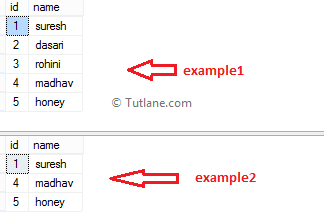# SQL ALL Operator

In SQL, the ALL operator is useful to return true when the given value matches with all the values in a single column set of values. The ALL operator in SQL is like an AND operator, and it will compare a value against all the values in a column.

## Syntax of SQL ALL Operator

Following is the syntax of defining an ALL operator in the SQL server.

SELECT column1, column2 FROM tablename WHERE column1 = ALL(SELECT column1 FROM tablename2)

To check all operators in SQL, execute the following script in the database to create tables.

create table example1(id int, name varchar(50))
insert into example1 values(1,'suresh'),(2,'dasari'),(3,'rohini'),(4,'madhav'),(5,'honey')

create table example2(id int, name varchar(50))
insert into example2 values(1,'suresh'),(4,'madhav'),(5,'honey')

select * from example1
select * from example2

Once we execute the above script, new tables example1 and example2 will create in the SQL database, and the result will be as shown below.Now our tables are ready, and we can write queries like as shown following to check ALL Operator.

## SQL ALL Operator Example1

Following is the example of using an all operator in the SQL server.

SELECT id,name FROM example1 WHERE id =ALL(SELECT Id FROM example2)

When we execute the above SQL all operator query, we will get the result below.If you observe the above result, we get 0 rows because the above query “id” in example1 table will compare with all ids in the example2 table, as shown below.

(example1:id1 = example2:id1)
AND (example1:id1 = example2:id4)
AND (example1:id1 = example2:id5)

## SQL ALL Operator Example2

Following is the example of using an all operator in sql server.

SELECT id,name FROM example1 WHERE id >=ALL(SELECT Id FROM example2)

When we execute the above SQL all operator query, we will get the result below.If you observe the above result, we got records because in the above query “id” column of example1 table, we will compare with all ids in the example2 table, like shown below.

(example1:id1 > example2:id1)
AND (example1:id1 > example2:id4)
AND (example1:id1 > example2:id5)

This is how we can use all operator in SQL server statements based on our requirements.